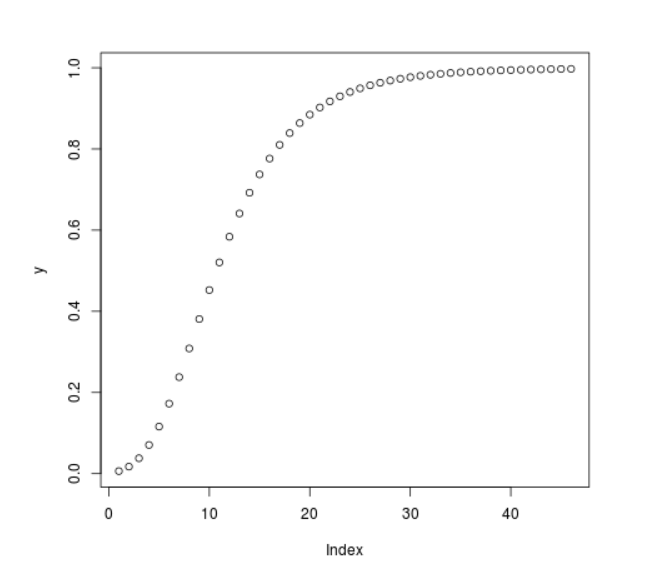Open In App

# Compute the value of CDF over Studentized Range Distribution in R Programming – ptukey() Function

`ptukey()` function in R Language is used to compute the value of Cumulative Distribution Function(CDF) over Studentized Range for a sequence of numeric values.

Syntax: ptukey(x, nmeans, df)

Parameters:
x: Numeric Vector
nmeans: Number of means
df: Degree of Freedom

Example 1:

 `# R Program to compute the value of``# CDF for Studentized Range`` ` `# Creating a sequence of Numeric values``x <``-` `seq(``1``, ``10``, by ``=` `1``)`` ` `# Calling ptukey() Function``y <``-` `ptukey(x, nmeans ``=` `7``, df ``=` `5``)``y`

Output:

```  0.01416051 0.22099429 0.54676395 0.76823006 0.88284988 0.93871676
 0.96637587 0.98063547 0.98833593 0.99268670
```

Example 2:

 `# R Program to compute the value of``# CDF for Studentized Range`` ` `# Creating a sequence of Numeric values``x <``-` `seq(``1``, ``10``, by ``=` `0.2``)`` ` `# Calling ptukey() Function``y <``-` `ptukey(x, nmeans ``=` `8``, df ``=` `7``)`` ` `# Plot a graph``plot(y)`

Output: